# PyVIMS¶

This notebook provide and a set of example of how to manipulate the Cassini VIMS data using the python pyvims module.

In :
import os

import wget

import matplotlib.pyplot as plt
from mpl_toolkits.basemap import Basemap

from pyvims import VIMS


## Get some data¶

To start this example your need to download some data. Here we will start with a cube (1487096932_1) calibrated with USGS-ISIS3 sofware and available on the vims.univ-nantes.fr data portal:

In :
vims_website = 'https://vims.univ-nantes.fr'

image_id = '1487096932_1'

for channel in ['vis', 'ir']:
for cube_type in ['C', 'N']:
fname = cube_type + image_id + '_' + channel + '.cub'

if not os.path.isfile('data/' + fname):

Downloading C1487096932_1_vis.cub


The location of the files can be specify with the root attribute.

In :
cube = VIMS('1487096932_1', root='data/')
cube

Out:
VIMS cube: 1487096932_1 [ISIS3]

The VIMS object provide a direct access to a subset of the cube metedata (extracted from the file header with the pvl module).

Here is a list of the metadata available about the capture time:

In :
# Acquisition start time (as datetime object)
print('Start:', cube.start)

# Acquisition stop time (as datetime object)
print('Stop:', cube.stop)

# Acquisition mid-time (as datetime object or %Y-%m-%dT%H:%M:%S.%f format)
print('Mid-Time:', cube.dtime, 'or', cube.time)

# Image year
print('Year:', cube.year)

# Image day of the year
print('DOY:', cube.doy)

# Image decimal year
print('Decimal year:', cube.year_d)

# Image date (as YYYY/MM/DD format)
print('Date:', cube.date)

Start: 2005-02-14 18:02:29.023000
Stop: 2005-02-14 18:07:32.930000
Mid-Time: 2005-02-14 18:05:00.976500 or 2005-02-14T18:05:00.976500
Year: 2005
DOY: 45
Decimal year: 2005.1205479452055
Date: 2005/02/14


## Extract data¶

Extract the spatial information for a specific band or wavelength:

In :
cube.getImg(band=167)

Out:
array([[-5.4426567e-04, -1.3694621e-04, -9.3489932e-04, ...,
6.3502282e-02,  5.7736006e-02,  5.2320253e-02],
[-5.4470141e-04, -1.4113497e-04, -1.4001154e-04, ...,
6.7836367e-02,  6.3464731e-02,  5.8084715e-02],
[ 2.5238303e-04,  2.5037612e-04, -1.4415705e-04, ...,
7.2807170e-02,  6.7784242e-02,  6.4127207e-02],
...,
[ 4.5216573e-05, -6.5096840e-04,  4.4637396e-05, ...,
-2.7215341e-04,  3.5355415e-04,  4.0521652e-05],
[ 4.0477469e-05,  4.0214873e-05, -3.0602355e-04, ...,
3.4900461e-04,  3.6208461e-05, -2.7794950e-04],
[-3.1504055e-04, -3.1299971e-04,  3.5281686e-05, ...,
3.1918044e-05,  3.1983360e-05,  3.2059252e-05]], dtype=float32)
In :
cube.getImg(wvln=2.03)

Out:
array([[-5.4426567e-04, -1.3694621e-04, -9.3489932e-04, ...,
6.3502282e-02,  5.7736006e-02,  5.2320253e-02],
[-5.4470141e-04, -1.4113497e-04, -1.4001154e-04, ...,
6.7836367e-02,  6.3464731e-02,  5.8084715e-02],
[ 2.5238303e-04,  2.5037612e-04, -1.4415705e-04, ...,
7.2807170e-02,  6.7784242e-02,  6.4127207e-02],
...,
[ 4.5216573e-05, -6.5096840e-04,  4.4637396e-05, ...,
-2.7215341e-04,  3.5355415e-04,  4.0521652e-05],
[ 4.0477469e-05,  4.0214873e-05, -3.0602355e-04, ...,
3.4900461e-04,  3.6208461e-05, -2.7794950e-04],
[-3.1504055e-04, -3.1299971e-04,  3.5281686e-05, ...,
3.1918044e-05,  3.1983360e-05,  3.2059252e-05]], dtype=float32)

Note: If the wavelength is provided, the closest corresponding band is selected (no interpolation between the bands).

To extract the 10 first wavelengths and the spectrum I/F at a specific location (sample/line):

In :
spec = cube.getSpec(S=20, L=15)

cube.wvlns[:10], spec[:10]

Out:
(array([0.35054, 0.35895, 0.36629, 0.37322, 0.37949, 0.3879 , 0.39518,
0.40252, 0.40955, 0.41731]),
array([0.05770097, 0.06194281, 0.06165274, 0.07216963, 0.06976949,
0.06189591, 0.05809197, 0.07248283, 0.06709387, 0.06832074],
dtype=float32))

To get the geographic coordinates:

In :
cube.getLon(S=20, L=15), cube.getLat(S=20, L=15)

Out:
(212.79308, -29.281103)

To get the illumination values:

In :
cube.getInc(S=20, L=15), cube.getEme(S=20, L=15), cube.getPhase(S=20, L=15)

Out:
(7.461604, 26.32574, 20.066418)

## Plot data¶

Plot image for a specific wavelength (ie auto-select the closest band):

In :
cube.plot_wvln(2.03)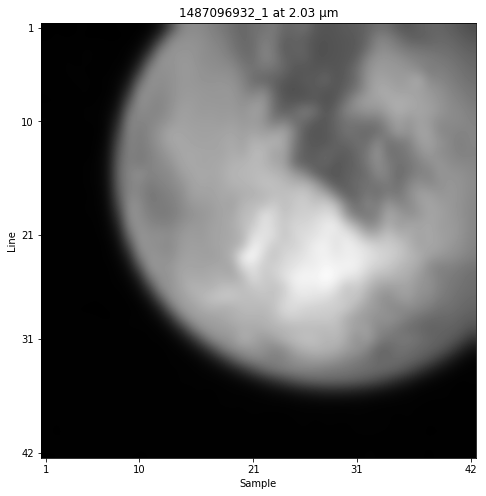In :
fig, axes = plt.subplots(1, 2, sharey=True, figsize=(12, 6))

cube.plot_wvln(2.03, ax=axes)
cube.plot_wvln(2.12, ax=axes)

axes.plot(20, 15, 'o')
axes.plot(28, 13, 'o')
axes.plot(20, 24, 'o');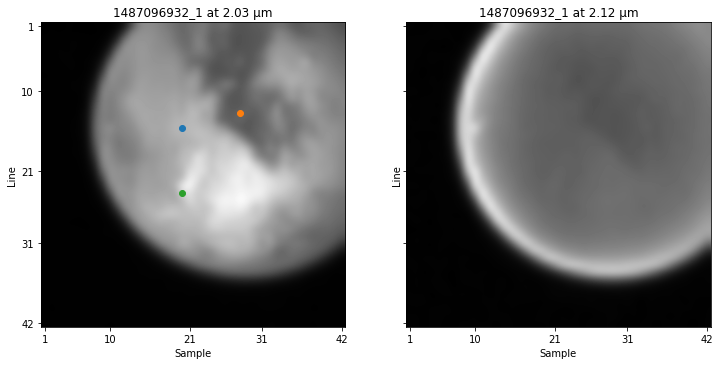Plot spectrum for a specific pixel (blue dot in the previous image):

In :
cube.plot_spectrum(S=20, L=15)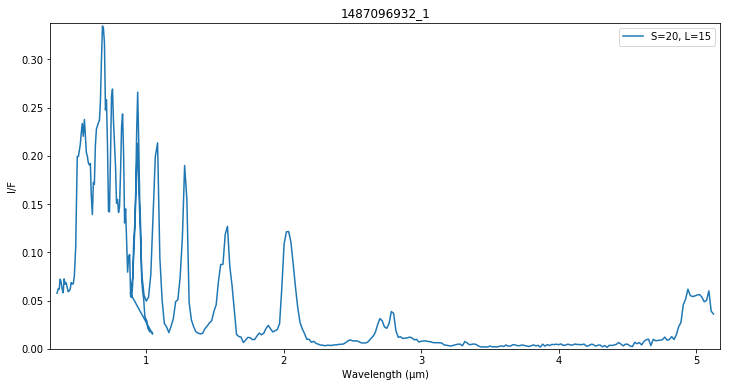Display multiple IR spectrum on the same plot:

In :
cube.plot_spectrum(S=[20, 28, 20], L=[15, 13, 24], yoffset=[0, .075, 0.15], vis=False)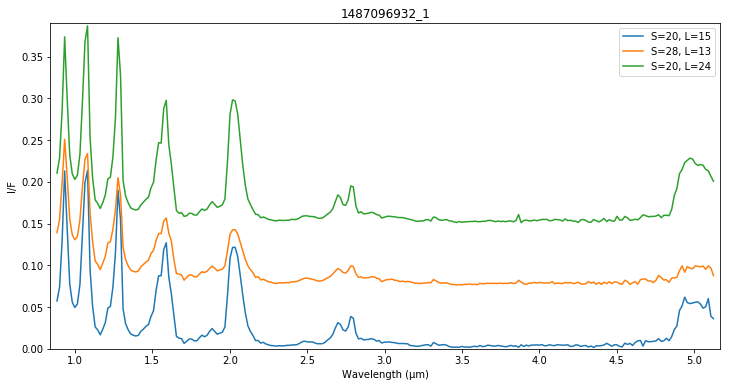## Mapping¶

Project the data on a map (longitude-latitude projection lonlat). The yellow dot corresponds to the sub-solar location, the yellow line represents the illumination great circle. The blue dot corresponds to the sub-spacecraft location, the blue line represents Cassini field of view. The cube data are interpolated within the image contour. The default wavelength used is 2.03 µm but can be changed with wvln attribute.

In :
cube.plot_map();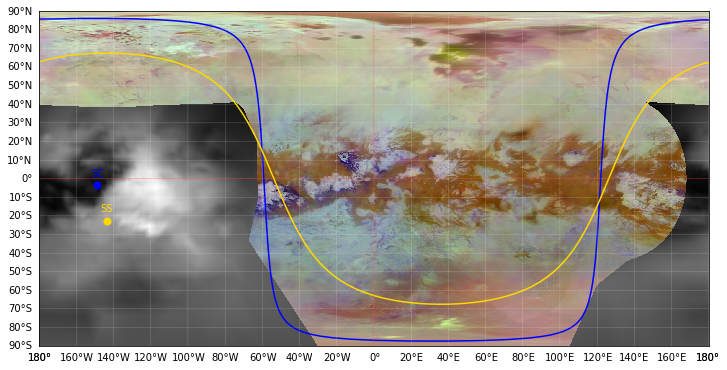Change the projection system to mollweide:

In :
cube.plot_map('mollweide');